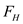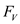# Consider a circular surface subjected to hydrostatic forces by a constant density liquid. If the magnitudes of the horizontal and vertical components of the resultant hydrostatic force are determined, explain how you would find the line of action of this force.

Question-AnswerCategory: Fluid MechanicsConsider a circular surface subjected to hydrostatic forces by a constant density liquid. If the magnitudes of the horizontal and vertical components of the resultant hydrostatic force are determined, explain how you would find the line of action of this force.

### Consider a circular surface subjected to hydrostatic forces by a constant density liquid. If the magnitudes of the horizontal and vertical components of the resultant hydrostatic force are determined, explain how you would find the line of action of this force.Hereis the angle made by the line of action,is the horizontal force component of hydrostatic force andis the vertical force component of hydrostatic force.